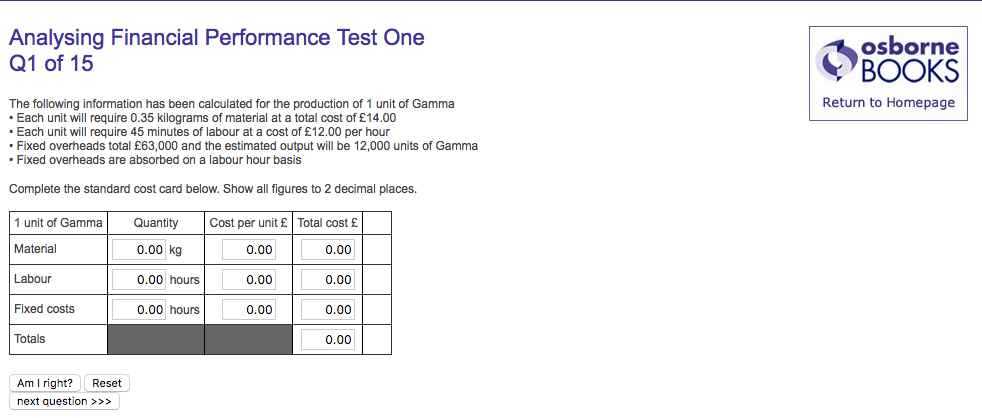# STANDARD COST CARD question...I get the materials row correct but the other two I think i understand but it tells me i'm wrong?!

• Material
Total cost is £14.00 for 0.35 kg. Therefore cost per unit = 14.00 / 0.35 = £40.00
Quantity = 0.35 kg
Cost per unit = £40.00
Total cost = £14.00

Labour
45 minutes = 3/4 of an hour = 0.75 hours. @ £12.00 per hour = £9.00
Quantity = 0.75 hours
Cost per unit = £12.00
Total cost = £9.00